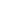Search found 3 results.Mathcad (1989)2 Mathematics MacOS

Mathcad is computer software primarily intended for the verification, validation, documentation and re-use of engineering calculations.MatLab (1986)13 Mathematics Windows MacOS

MatLab is a high speed, interactive programming utility for manipulating, calculating, and plotting complicated mathematical equations. It is considered easy to use for those familiar with typical algebra equations.StatWorks (1985)0 Mathematics MacOS

StatWorks, from Cricket Software, is a very powerful statistics software package for the Macintosh designed for a wide variety of applications. It enables all who work with numbers to do statistical computations without spending hours learning "high level" languages or going through several stages to compile analysis.